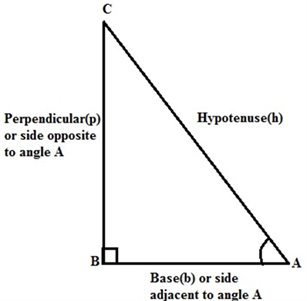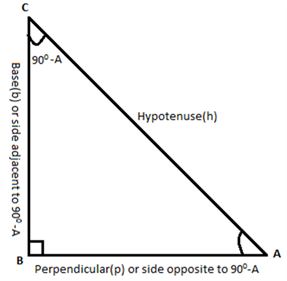# Trigonometric Ratios of Complementary Angles

In Mathematics, the complementary angles are the set of two angles such that their sum is equal to 90°. For example, 30° and 60° are complementary to each other as their sum is equal to 90°. In this article, let us discuss in detail about the complementary angles and the trigonometric ratios of complementary angles with examples in a detailed way.

## Complementary Angles Definition

The two angles, say ∠X and ∠Y are complementary if,

∠X + ∠Y = 90°

In such a condition ∠X is known as the complement of ∠Y and vice-versa.

In a right angle triangle, as the measure of the right angle is fixed, the remaining two angles always form the complementary as the sum of angles in a triangle is equal to 180°.

## Finding Trigonometric Ratios of Complementary Angles

Assume a triangle ∆ABC, which is right-angled at B.∠A and ∠C form a complementary pair.

⇒ ∠A + ∠C = 90°

The relationship between the acute angle and the lengths of sides of a right-angle triangle is expressed by trigonometric ratios. For the given right angle triangle, the  trigonometric ratios of ∠A is given as follows:

sin A = BC/AC

cos A = AB/AC

tan A =BC/AB

csc A = 1/sin A = AC/BC

sec A =1/cos A = AC/AB

cot A = 1/tan A = AB/BC

The trigonometric ratio of the complement of ∠A. It means that the ∠C can be given as 90° – ∠AAs ∠C = 90°- A (A is used for convenience instead of ∠A ), and the side opposite to 90° – A is AB and the side adjacent to the angle 90°- A is BC as shown in the figure given above.

Therefore,

sin (90°- A) = AB/AC

cos (90°- A) = BC/AC

tan (90°- A) = AB/BC

csc (90°- A) =1/sin (90°- A) = AC/AB

sec (90°- A) = 1/cos (90°- A) = AC/BC

cot (90°- A) = 1/tan (90°- A)  = BC/AB

Comparing the above set of ratios with the ratios mentioned earlier, it can be seen that;

sin (90°- A) = cos A ; cos (90°- A) = sin A

tan (90°- A) = cot A; cot (90°- A) = tan A

sec (90°- A) = csc A; csc (90°- A) = sec A

These relations are valid for all the values of A that lies between 0° and 90°.

Summary:

• Sin of an angle = Cos of its complementary angle
• Cos of an angle = Sin of its complementary angle
• Tan of an angle = Cot of its complementary angle

### Trigonometric Ratios of Complementary Angles Examples

To have a better insight on trigonometric ratios of complementary angles consider the following example.

Example:

If A, B and C are the interior angles of a right-angle triangle, right-angled at B then find the value of A, given that tan 2A = cot(A – 30°) and 2A is an acute angle.

Solution:

Using the trigonometric ratio of complementary angles,

cot (90°- A) = tan A

From this ratio, we can write the above expression as:

⇒ tan 2A = cot (90°- 2A) ….(1)

Given expression is tan 2A = cot (A – 30°) …(2)

Now, equate the equation (1) and (2), we get

cot (90°- 2A) = cot (A – 30°)

⇒ 90°- 2A = A – 30°

⇒3A = 90° + 30°

⇒3A = 120°

⇒A = 120°/ 3

⇒ A = 40°

Thus, the measure of the acute angle A can be easily calculated by making use of trigonometry ratio of complementary angles.

## Video Lesson

### Trigonometric Ratios of Compound AnglesTo know more about trigonometric ratios of complementary angles and its applications, download BYJU’S – The Learning App.

Quiz on Trigonometric Ratios of Complementary Angles